# MATHEMATICS —2004 (Set III— Delhi)

Except for the following questions, all the remaining questions have been asked in Set I and Set II.

Q. 2. The 7 th term of an A.P. is -4 and its 13th term is -16. Find the A.P.

Ans.(8, 6, 4, 2, 0, -2, -4, ..)Q. 5. Solve for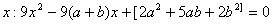Ans.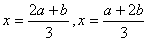Q. 7. A loan of Rs.21,200 has to be repaid in two equal annual instalments. If the interest is charged at the rate of12% per annum, compounded annually, find the amount of each instalment.

Ans.(Rs.12,544)Q. 8. A radio is available for Rs.1,500 cash or Rs.350 as cash down payment followed by four equal monthly instalments of Rs.300. Find the rate of interest charged under the instalment scheme.

Ans.(21.42% approx)

Q. 11. A solid metall1c sphere of diameter 21 cm is melted and recasted into a number of smaller cones, each of diameter 3.5 cm and height 3 cm. Find the number of cones so formed.

Ans.( 504 cones)

Q. 14. Solve for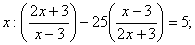given that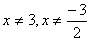.

Ans.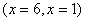Q. 20. A bag contains 6 black, 7 red and 2 white balls. A ball is drawn from the bag at random. Find the probability that the ball drawn is (i) red (ii) black or white (iii) not black.

Ans.(7/15, 8/15, 3/5)

Q. 21. Madan Gopal has an annual income of Rs. 3,60,000 (exclusive of HRA). He contributes Rs. 6,000 per month towards his GPF and pays an annual LIC premium of Rs. 15,000. He has invested Rs. 10,000 in N.S.C's. He pays Rs. 5,500 as income tax per month for the first 11 months. Find his income tax liability for the last month of the financial year.

Use the following for calculating income tax:

 (a) Standard Deduction Gross income Deduction (i) Upto Rs.75,000 (ii) From Rs.75,001 to Rs.5,00,000 (iii) More than Rs,5,00,000 40% of gross income Rs.30,000 Rs.20,000 (b) Rates of Taxes Slab Income tax (i) Upto Rs. 50,000 (ii) From Rs. 50,001 to Rs. 60,000 (iii) From Rs. 60,001 to Rs. 1,50,000 (iv) Rs. 1.50,000 and above No tax 10% of the amount exceeding Rs.50,000 Rs.1,000+20% of the amount Exceeding Rs.60,000 Rs.19,000+30% of the amount Exceeding Rs.1,50,000 (c) Rebate in Tax (i) 20% of the savings subject to a maximum of Rs. 14,000 if the gross income is upto Rs. 1,50,000. (ii)15% of the savings subject to a maximum of Rs. 10,500 if the gross income is from Rs. 1,50,001 to Rs. 5,00,000.

Ans.(Rs.10,300)

Maths 2004 Question Papers Class X
CBSE 2004 Question Papers Class X